# 深度学习速查表 Star

## 神经网络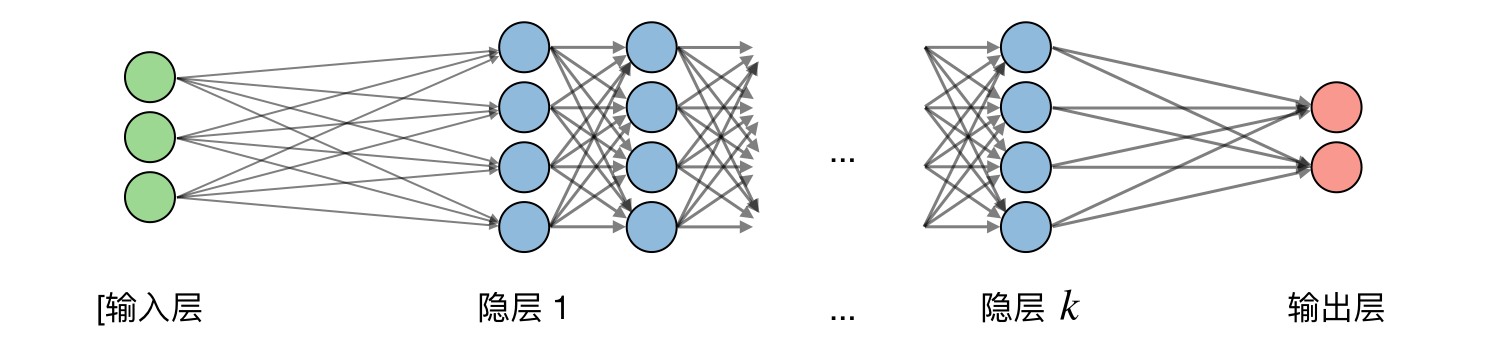$\boxed{z_j^{[i]}={w_j^{[i]}}^Tx+b_j^{[i]}}$

 逻辑函数(Sigmoid) 双曲正切函数(Tanh) 线性整流函数(ReLU) 带泄露线性整流函数(Leaky ReLU) $g(z)=\displaystyle\frac{1}{1+e^{-z}}$ $g(z)=\displaystyle\frac{e^{z}-e^{-z}}{e^{z}+e^{-z}}$ $g(z)=\textrm{max}(0,z)$ $g(z)=\textrm{max}(\epsilon z,z)$ with $\epsilon\ll1$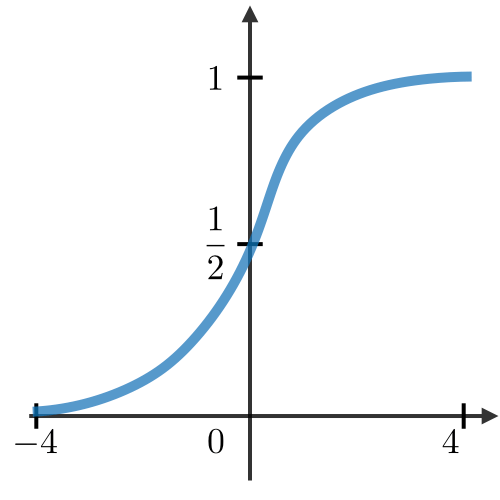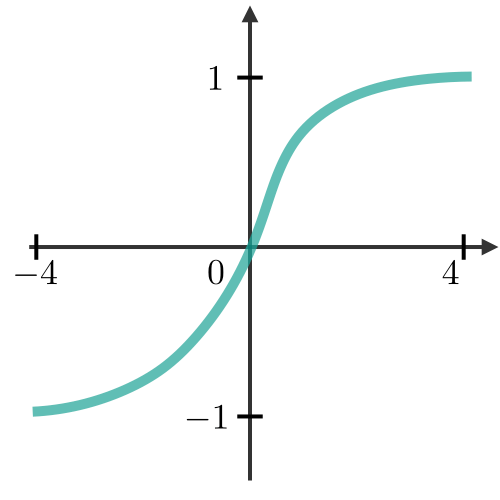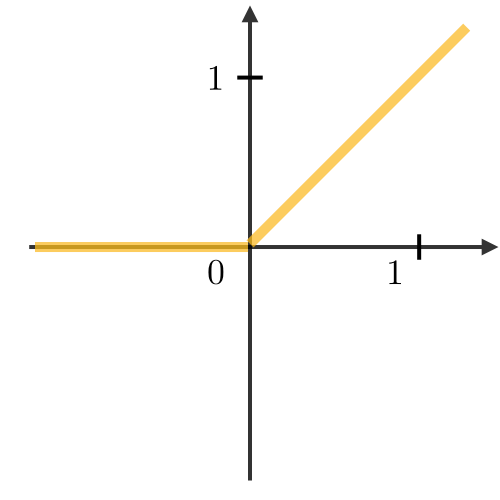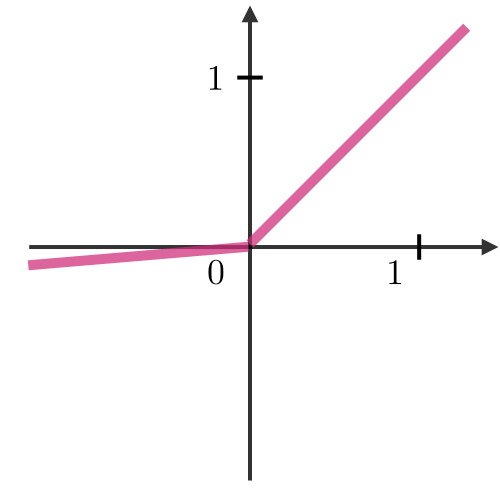$\boxed{L(z,y)=-\Big[y\log(z)+(1-y)\log(1-z)\Big]}$

$\boxed{\frac{\partial L(z,y)}{\partial w}=\frac{\partial L(z,y)}{\partial a}\times\frac{\partial a}{\partial z}\times\frac{\partial z}{\partial w}}$

$\boxed{w\longleftarrow w-\alpha\frac{\partial L(z,y)}{\partial w}}$

- 第一步 : 分出第一批次的训练数据。
- 第二步 : 通过前向传播来得到相关损失。
- 第三步 : 通过反向传播损失来得到梯度。
- 第四步 : 利用梯度更新网络的权重。

## 卷积神经网络

$\boxed{N=\frac{W-F+2P}{S}+1}$

$\boxed{x_i\longleftarrow\gamma\frac{x_i-\mu_B}{\sqrt{\sigma_B^2+\epsilon}}+\beta}$

## 递归神经网络

 输入门 忘记门 门控 输出门 要不要写入单元？ 要不要清空单元？ 在单元写入多少？ 从单元泄露多少？

LSTM ― 长短期记忆网络是递归神经网络的一种，它可以通过增加“遗忘”门控来避免梯度消失问题。

## 强化学习和控制

### 定义

- $\mathcal{S}$ 是状态的集合
- $\mathcal{A}$ 是动作的集合
- $\{P_{sa}\}$ 是对于 $s$ 属于 $\mathcal{S}$ 并且 $a$ 属于 $\mathcal{A}$ 的状态转换概率
- $\gamma\in[0,1[$ 是折扣系数
- $R:\mathcal{S}\times\mathcal{A}\longrightarrow\mathbb{R}$ 或 $R:\mathcal{S}\longrightarrow\mathbb{R}$ 是算法希望最大化的回报函数

$\boxed{V^\pi(s)=E\Big[R(s_0)+\gamma R(s_1)+\gamma^2 R(s_2)+...|s_0=s,\pi\Big]}$

$\boxed{V^{\pi^*}(s)=R(s)+\max_{a\in\mathcal{A}}\gamma\sum_{s'\in S}P_{sa}(s')V^{\pi^*}(s')}$

$\boxed{\pi^*(s)=\underset{a\in\mathcal{A}}{\textrm{argmax}}\sum_{s'\in\mathcal{S}}P_{sa}(s')V^*(s')}$

1) 首先我们初始化值：

$\boxed{V_0(s)=0}$

2) 我们通过之前的值进行迭代：

$\boxed{V_{i+1}(s)=R(s)+\max_{a\in\mathcal{A}}\left[\sum_{s'\in\mathcal{S}}\gamma P_{sa}(s')V_i(s')\right]}$

$\boxed{P_{sa}(s')=\frac{\#\textrm{状态 }s\textrm{ 下进行动作 }a\textrm{ 并且进入状态 }s‘\textrm{ 的次数}}{\#\textrm{状态 }s\textrm{ 下进行动作 }a\textrm{ 的次数}}}$

$Q$ 学习 ― $Q$ 学习是一种 $Q$ 的无模型(model-free)估计，如下所示：

$\boxed{Q(s,a)\leftarrow Q(s,a)+\alpha\Big[R(s,a,s')+\gamma\max_{a'}Q(s',a')-Q(s,a)\Big]}$